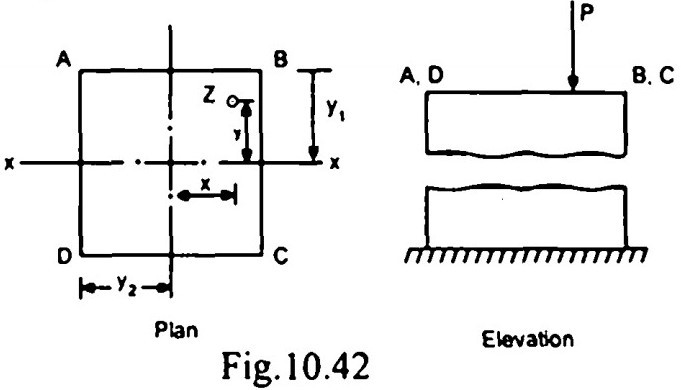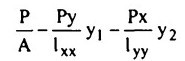Search

# Strength of Materials(221-240)

Updated: Apr 28, 2020

221.The cantilever frame shown in Fig.is supported by vertical links at B and C

and carries loads as shown. The force in the bar AE is zero

222. If the loads and reactions of the beam shown in Fig. 10.35 are as given in the

figurethe thrust diagram on the section of the223. For the beam shown in Fig. 10.37, the

maximum positive bending moment is

equal to negative bending moment.The value of L1 is L/22

224.For the shear force diagram shown in Fig. the loaded beam will be225. A simply supported beam of span L carries a concentrated load W at mid-span. If the width b of the beam is constant and its depth is varying throughout the span, then what should be its mid-span depth, when design stress is f ?226. For the cantilever beam shown in Fig. which one of the following pairs is

correctly matched ?227. A cantilever carries a load P at C as shown in Fig.228. A cantilever of constant depth carries a uniformly distributed load on the whole span. To make the maximum stress at all sections the same, the breadth of the section at a distance x from the free end should be proportional to

X²

229.For a circular column having its ends hinged, the slendemess ratio is 160. The

1/6 ratio of the column is 40

230.A hollow circular column of internal diameter d and external diameter 1.5 d is

subjected to compressive load. The maximum distance of the point of application of load from the centre for no tension is

13d/48

231.

Rectangular- Rhombus I-section - Rhombus Hollow circular- Circle Square - Square

232. A column ABCD (2y, x 2y2) of rectangular section carries a load P at Z

having the coordinates (x, y) as shown in Fig.If the compressive stresses are taken as positive and area A=2y x 2y = 4yy and

the moment of intertia about x and y axis being lxx and lyy respectively, then the

stress at the corner D is233. A simply supported beam of span 1 and flexural rigidity EI, carries a unit point

load at its centre. The strain energy in the beam due to bending is234. Two closed-coit springs of stiffness s and 2s are arranged in'series in one case and in parallel in the other case. The ratio of stiffness of springs connected in series to parallel is 2/9

235.

a) Lame's constants : Thick cylinder

b) Macaulay's method: deflection of beams

c) Euler's method: Theory of columns

236. Two closed thin vessels, one cylindrical and the other spherical with equal internal diameter and wall thickness are subjected to equal internal fluid pressure. The ratio of hoop stresses in the cylindrical to that of spherical vessels is

2.0

237. A round steel bar of overall length 40 cm consists of two equal portions of 20 cm each having diameters of 10 cm and 8 cm respectively. If the rod is subjected to a tensile load of 10 tonnes, the elongation will be given by (E = 2x 10 kg/cm²)238. A copper bar of 25 cm length is fixed by means of supports at its ends. Supports can yield (total) by 0.01 cm. If the temperature of the bar is raised by 100°C, then the stress induced in the bar for ∝𝒸 = 20 x lO⁻⁶/°C and Ec = 1 x 10 kg/cm² will be

16x10² kg /cm²

239

A. Moment of inertia - Modulus of rupture B. Elongation - Tensile stress C. Neutral axis - Zero longitudinal stress D. Top fibre - Zero shear stress

240. According to the Distortion Energy Theory, failure will not occur when

(symbols have the usual meaning)